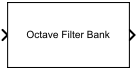# Octave Filter Bank

Octave-band and fractional octave-band filter bank

• Library:
• Audio Toolbox / Filters

•## Description

The Octave Filter Bank block decomposes a signal into octave or fractional-octave subbands. An octave-band is a frequency band where the highest frequency is twice the lowest frequency. Octave-band and fractional octave-band filters are commonly used to mimic how humans perceive loudness.

## Ports

### Input

expand all

• Vector input –– The block treats the input as a single channel.

• Matrix input –– The block treats each column of the input as an independent channel.

Data Types: `single` | `double`

### Output

expand all

The Octave Filter Bank block outputs a signal with the same data type as the input signal. The shape of the output depends on the shape of the input, the number of filters in the bank, and whether or not you enable the Bands as separate output ports parameter.

If F is the number of filters in the bank, and the input signal is an L-by-C matrix, then the block returns an L-by-F-by-C array. If C is 1, then the block outputs a matrix.

• Vector input –– When you provide a vector input, the block outputs an L-by-F matrix, where L is the number of elements in the vector and F is the number of filters in the bank.

• Matrix input –– When you provide a matrix input, the block outputs a 3-D array with size L-by-F-by-C, where C is the number of channels in the matrix input.

Note

When you enable the Bands as separate output ports parameter, each output is the same size as the input.

Data Types: `single` | `double`

## Parameters

expand all

Filter bandwidth in octaves, specified as `1 octave`, ```2/3 octave```, `1/2 octave`, `1/3 octave`, `1/6 octave`, `1/12 octave`, ```1/24 octave```, `1/48 octave`.

Frequency range of the filter bank in Hz, specified as a two-element row vector of positive monotonically increasing values. The block places filter bank center frequencies according to the Bandwidth (octaves), Reference frequency (Hz), and Octave ratio base parameters. Filters that have a center frequency outside of Frequency range (Hz) are ignored.

Reference frequency of the filter bank in Hz, specified as a positive integer scalar. The reference frequency defines one of the center frequencies. All other center frequencies are set relative to the reference frequency.

Order of the octave filters, specified as a positive even integer. The filter order applies to each individual filter in the bank.

Note

The default filter order for the `octaveFilterBank` object is `2`.

Octave ratio base, specified as ```Base ten (ANSI S1.11 preferred)``` or `Base two (musical scale)`. The octave ratio base determines the distribution of the center frequencies of the octave filters. The ANSI S1.11 standard recommends base 10. Base 2 is popular for music applications. Base two (musical scale) defines an octave as a factor of 2, and Base ten (ANSI S1.11 preferred) defines an octave as a factor of 10 0.3.

When you select this parameter, the block inherits its sample rate from the input signal. When you clear this parameter, you specify the sample rate in the Input sample rate (Hz) parameter.

When you select this parameter, the block accepts the sample rate from the user.

#### Dependencies

To enable this parameter, clear the Inherit sample rate from input parameter.

When you select this parameter, the block provides an output port for each filter in the bank. Each output port is labeled with the center frequency of the filter and has a size identical to the input signal.

• `Code generation` –– Simulate the model using generated C code. The first time you run a simulation, Simulink® generates C code for the block. The C code is reused for subsequent simulations as long as the model does not change. This option requires additional startup time.

• `Interpreted execution` –– Simulate the model using the MATLAB® interpreter. In this mode, you can debug the source code of the block.

Octave filters are best understood when viewed on a logarithmic scale, which models how the human ear weights the spectrum. The block uses a 2048-point FFT to calculate the magnitude response. The filter bank's response is displayed on a log-frequency scale with a legend to indicate the center frequency of each filter.

## Block Characteristics

 Data Types `double` | `single` Direct Feedthrough `no` Multidimensional Signals `no` Variable-Size Signals `yes` Zero-Crossing Detection `no`

expand all

## Algorithms

expand all

The Octave Filter Bank block is implemented as a parallel structure of octave filters. Individual octave filters are designed as described by `octaveFilter`. By default, the octave filter bank center frequencies are placed as specified by the `ANSI S1.11-2004` standard. You can modify the filter placements using the Bandwidth (octaves), Frequency range (Hz), Reference frequency (Hz), and Octave ratio parameters.

 , Sophocles J. Introduction to Signal Processing. Englewood Cliffs, NJ: Prentice Hall, 2010.

 Acoustical Society of America. American National Standard Specification for Octave-Band and Fractional-Octave-Band Analog and Digital Filters: ANSI S1.11-2004. Melville, NY: Acoustical Society of America, 2009.

## SupportGet trial now